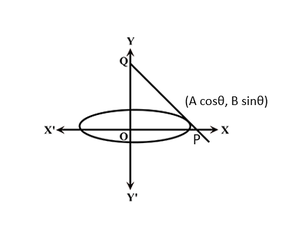# Minimum area of the triangle formed by any tangent to an ellipse with the coordinate axes

• Last Updated : 07 Apr, 2021

Given two integers A and B, representing the length of the semi-major and semi-minor axis of an ellipse with the equation (x2 / A2) + (y2 / B2) = 1, the task is to find the minimum area of the triangle formed by any tangent to the ellipse with the coordinate axes.

Examples:

Input: A = 1, B = 2
Output: 2

Input: A = 2, B = 3
Output: 6

Approach:The idea is based on the observation that the equation of a tangent at coordinate (A * cosθ, B * sinθ) on the ellipse in the figure shown above is:

(x * cosθ / A) + (y * sinθ / B) = 1

The coordinates of P and Q are (A / cosθ, 0) and (0, B / sinθ) respectively.
Area of triangle formed by the tangent to the ellipse and the coordinate axes is given by:

Area of ΔOPQ = 0.5 * base * height = 0.5 * (A / cosθ) * (B / sinθ) = (A * B) / (2 * sinθ * cosθ) = (A * B) / sin2θ

Therefore, Area = (A * B) / sin2θ — (1)

To minimize the area, the value of sin2θ should be maximum possible, i.e. 1.
Therefore, the minimum area possible is A * B.

Below is the implementation of the above approach:

## C++

 `// C++ program for the above approach` `#include ` `using` `namespace` `std;`   `// Function to find the minimum area` `// of triangle formed by any tangent` `// to ellipse with the coordinate axes` `void` `minimumTriangleArea(``int` `a, ``int` `b)` `{` `    ``// Stores the minimum area` `    ``int` `area = a * b;`   `    ``// Print the calculated area` `    ``cout << area;` `}`   `// Driver Code` `int` `main()` `{` `    ``int` `a = 1, b = 2;` `    ``minimumTriangleArea(a, b);`   `    ``return` `0;` `}`

## Java

 `// Java program for the above approach` `class` `GFG{`   `// Function to find the minimum area` `// of triangle formed by any tangent` `// to ellipse with the coordinate axes` `static` `void` `minimumTriangleArea(``int` `a, ``int` `b)` `{` `    `  `    ``// Stores the minimum area` `    ``int` `area = a * b;`   `    ``// Print the calculated area` `    ``System.out.println(area);` `}`   `// Driver Code` `public` `static` `void` `main(String[] args)` `{` `    ``int` `a = ``1``, b = ``2``;` `    `  `    ``minimumTriangleArea(a, b);` `}` `}`   `// This code is contributed by AnkThon`

## Python3

 `# Python3 program for the above approach`   `# Function to find the minimum area` `# of triangle formed by any tangent` `# to ellipse with the coordinate axes` `def` `minimumTriangleArea(a, b):` `    `  `    ``# Stores the minimum area` `    ``area ``=` `a ``*` `b`   `    ``# Print the calculated area` `    ``print``(area)`   `# Driver Code` `a ``=` `1` `b ``=` `2`   `minimumTriangleArea(a, b)`   `# This code is contributed by rohitsingh07052`

## C#

 `// C# program for the above approach` `using` `System;`   `class` `GFG{` `    `  `// Function to find the minimum area` `// of triangle formed by any tangent` `// to ellipse with the coordinate axes` `static` `void` `minimumTriangleArea(``int` `a, ``int` `b)` `{` `    `  `    ``// Stores the minimum area` `    ``int` `area = a * b;`   `    ``// Print the calculated area` `    ``Console.WriteLine(area);` `}`   `// Driver Code` `public` `static` `void` `Main()` `{` `    ``int` `a = 1, b = 2;` `    `  `    ``minimumTriangleArea(a, b);` `}` `}`   `// This code is contributed by ukasp`

## Javascript

 `// JavaScript program for the above approach`   `// Function to find the minimum area` `// of triangle formed by any tangent` `// to ellipse with the coordinate axes` `function` `minimumTriangleArea(a, b)` `{` `    `  `    ``// Stores the minimum area` `    ``var` `area = a * b`   `    ``// Print the calculated area` `   ``console.log(area)` `    `  `}`   `// Driver Code` `var` `a = 1` `var` `b = 2`   `minimumTriangleArea(a, b)`   `// This code is contributed by AnkThon`

Output

`2`

Time Complexity: O(1)
Auxiliary Space: O(1)

My Personal Notes arrow_drop_up
Recommended Articles
Page :